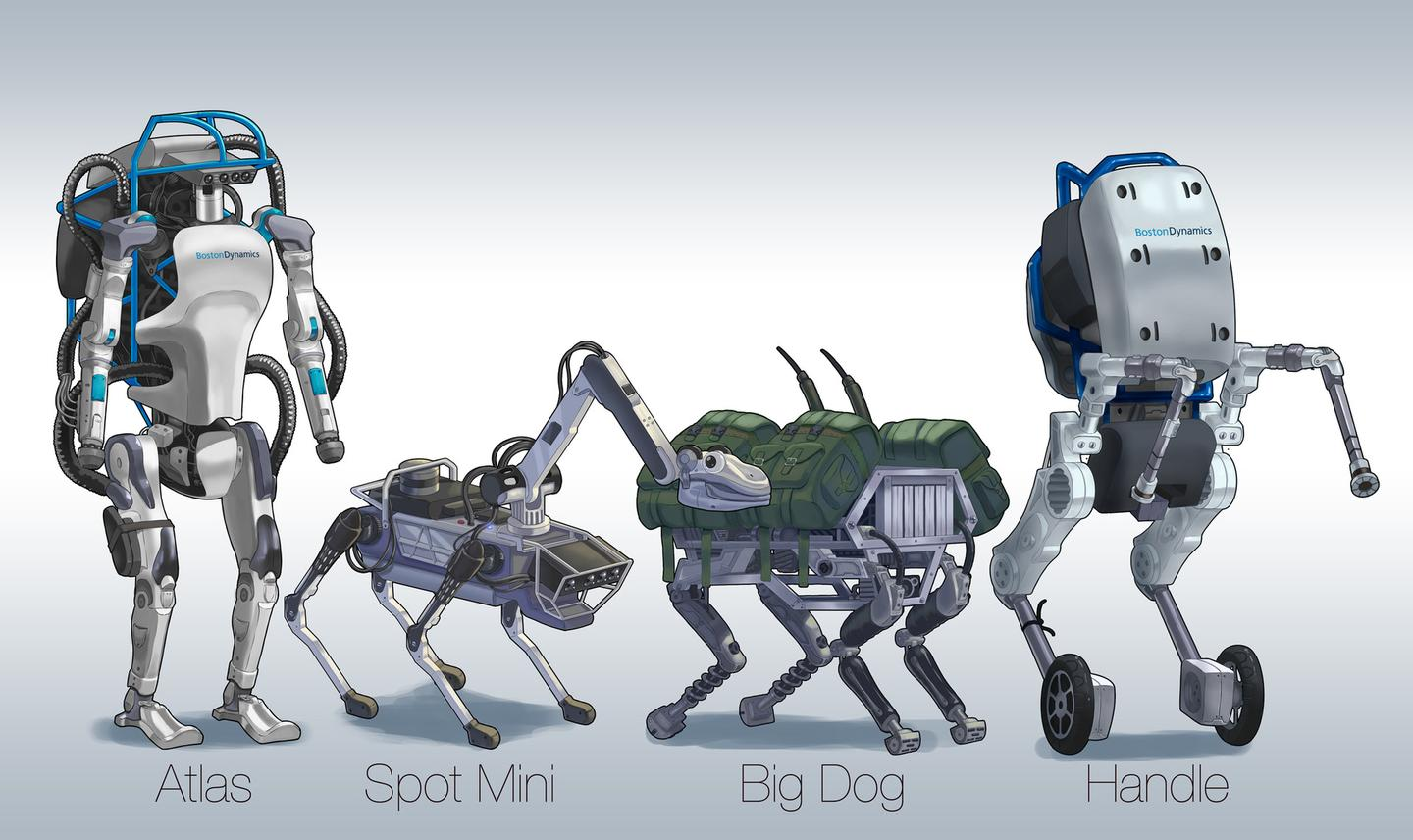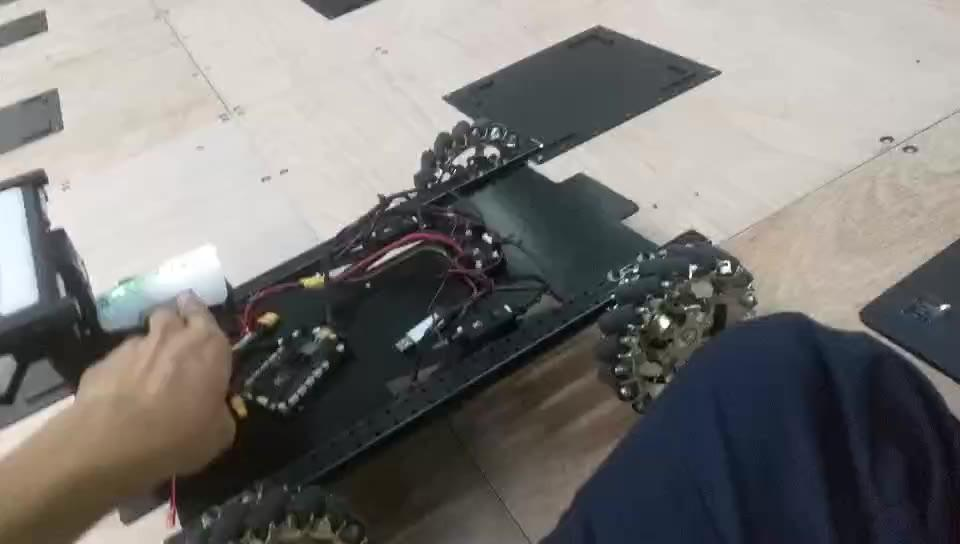• 运动学
• 动力学
• 控制学

## 关于这篇文章

• 微分方程(Differential equation)
• 控制目标与误差空间(Control goal and error space)
• 线性控制(Linear control)
• 基于模型的非线性控制(一) (Model-based nonlinear control)

• 控制规律分解(Control-law partitioning)
• 基于模型的非线性控制(二) (Model-based nonlinear control)
• 轨迹生成(Trajectory generation)
• 轨迹跟踪控制(Trajectory following control)

## 背景知识

• 基本的微分方程的概念, 不需要会求解:的解是• 基本力矩及转动惯量的概念(在某些例子会用到)

## 控制目标与误差空间(不考虑正负的问题)

•时, 特征方程有两个不等的实数根, 系统主要受摩擦力影响, 会缓慢回到平衡点而不发生震荡, 微分方程的解是:, 系数通过两个附加条件可以算出( 通常是初始位置和初始速度), 图片及Matlab程序(可以更改各个参数及初始位置试试):

%set parameter
m = 1;
b = 5;
k = 6;

%set the initial pos
x0 = -1;

%calculate the s1 s2
s1 = -b/2*m + sqrt(b^2 - 4*m*k)/2*m;
s2 = -b/2*m - sqrt(b^2 - 4*m*k)/2*m;

%set the po and calculate the c1 c2 from inital pos
c1 = x0*s2/(s2-s1);
c2 = x0*s1/(s1-s2);

t = 0:0.1:3;
x = c1*exp(s1*t)+c2*exp(s2*t);

plot(t,x);
xlabel('t','FontSize',16);
ylabel('x(t)','FontSize',16);
•时, 特征方程有两个不等的复根, 系统主受到弹性力的影响, 会出现震荡, 微分方程的解也是是:, 但是因为包含虚数, 比较复杂, 经过一系列(欧拉公式)的变换后, 可以得到, 其中, 由于情况比较复杂, 而且不是我们要讨论的重点, 所以省略了很多东西, 轨迹图像和Matlab代码(只算了一下m=1, b=1, k=1的情况):

m=1, b=1, k=1初始位置为-0.6时物块被释放的轨迹

t = 0:0.1:20;
x = 2*sqrt(3)/3*exp(-t/2).*cos(sqrt(3)/2*t - 2*pi/3);
plot (t,x);
xlabel('t','FontSize',16);
ylabel('x(t)','FontSize',16);
•时, 特征方程有两个相等的实数根, 将以最短的时间回到平衡位置,这时系统处于临界阻尼状态, 微分方程的解是:, 图像和Matlab代码(可以调整参数试试):

%set the fixed parameter
m = 1;
b = 4;
k = b^2/(4*m);

%set the intial pos and calculate the c1 c2 from inital pos
x0 = -0.6;
c1 = x0;
c2 = x0;

t = 0:0.1:5;
x = (c1+c2*t).*exp(-b*t/2*m);

plot(t,x);
xlabel('t','FontSize',16);
ylabel('x','FontSize',16);“设计一个控制器, 使整个系统在任何时刻满足微分方程而且, 这样无论初始误差等于多少, 它都会以最快的速度到达0, 而不发生震荡.

1D四轴飞行器

## 基于模型的非线性控制, 然后我们就能按照之前的思路, 找到另 整个系统满足.

## 参考文献

Craig, John J. 机器人学导论. China Machine Press, 2005.

Craig, J. J. (2005).Introduction to robotics: mechanics and control(Vol. 3, pp. 48-70). Upper Saddle River, NJ, USA:: Pearson/Prentice Hall.

Coursera Robotics: Aerial Robotics 的部分课程

## 预告自动小车底盘https://www.zhihu.com/video/1018597116473614336